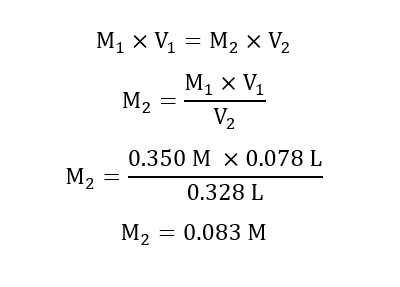# Calculate the molarity of 78.0 mL of 0.350 M NaOH diluted to 0.250 L with water. Answer in M, not in scientific notation.

Question
1 views

Calculate the molarity of 78.0 mL of 0.350 M NaOH diluted to 0.250 L with water. Answer in M, not in scientific notation.

check_circle

Step 1

Given:

Initial concentration before dilution M1 = 0.350 M

Initial volume V1 = 78.0 mL = 0.078 L.

Final volume V2 = 0.0780 L + 0.250 L = 0.328 L

Step 2

Calculation for Concentration(molarity) after dilution M2:...

### Want to see the full answer?

See Solution

#### Want to see this answer and more?

Solutions are written by subject experts who are available 24/7. Questions are typically answered within 1 hour.*

See Solution
*Response times may vary by subject and question.
Tagged in